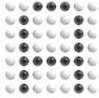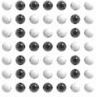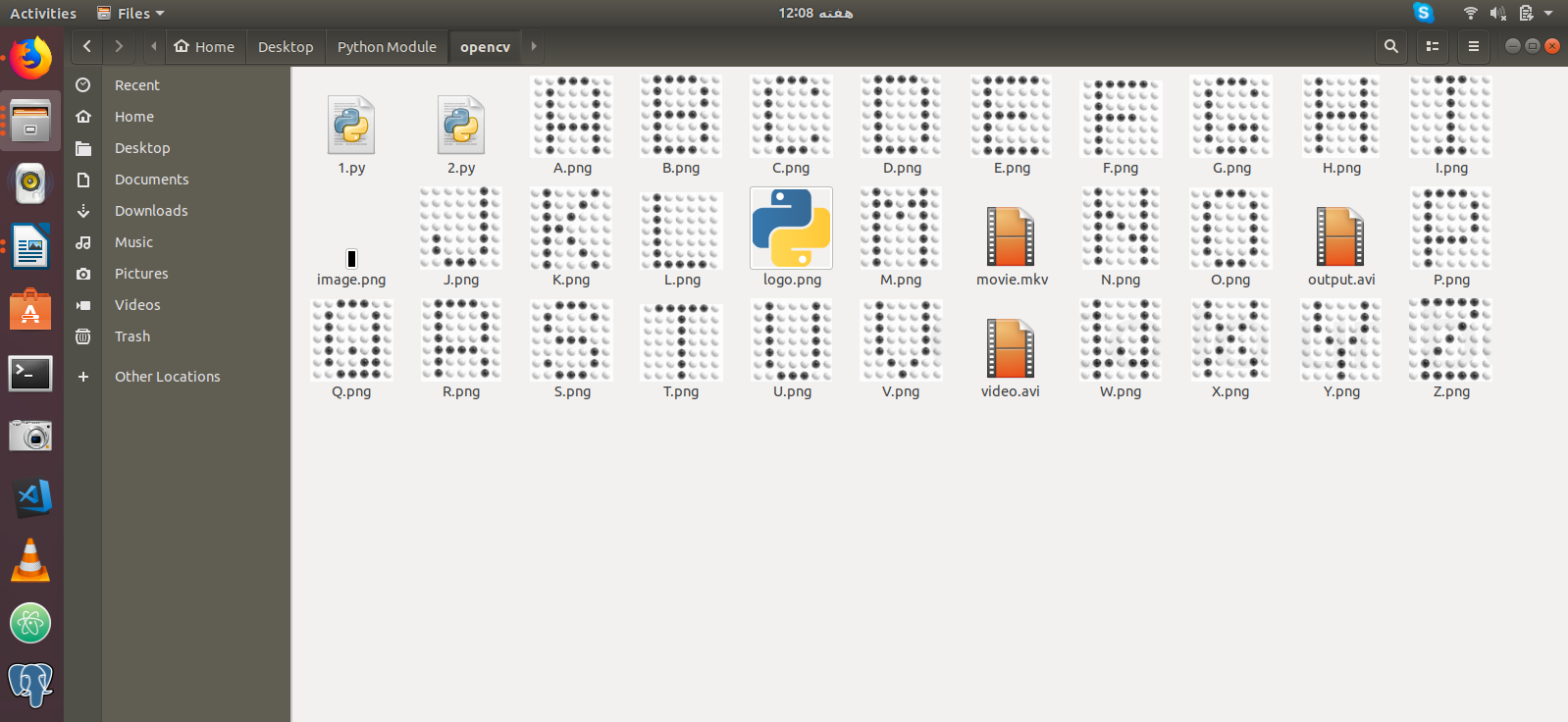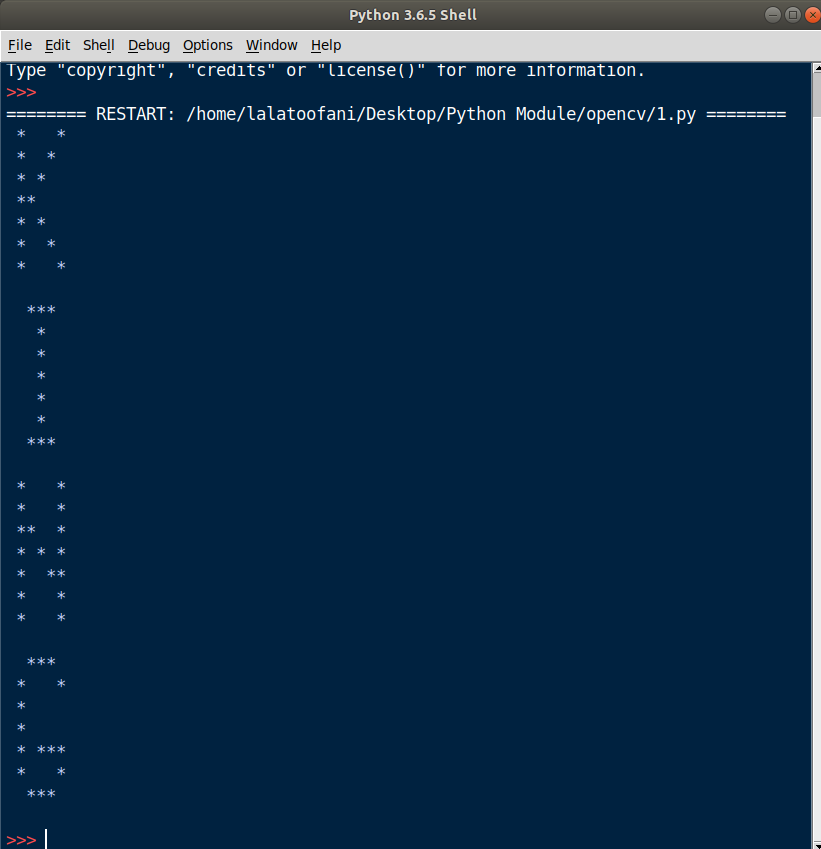## How Pixels Works in Python

##### In this part of Learning Python we Cover How Pixels Works In Python
Written by |10-May-2019 | 0 Comments | 327 Views

Now we will see some more aspects of python OpenCV. So, let’s start with writing the video. We have seen how we can start the web camera and visualize the image. However, sometimes we have to store the video in a format that we can watch it later. For example, if a company is doing some observation via webcam or IP cam. The person in front of a computer cannot monitor the footage all the time. In such cases, we store the video in a local computer for later usage. Now we will see how we can save the video. Following is the code:

`import numpy as np`
`import cv2`

`cap = cv2.VideoCapture(0)`

`fourcc = cv2.VideoWriter_fourcc(*'MJPG')`
`out = cv2.VideoWriter('video.avi', fourcc, 20.0, (640,480))`

`while(cap.isOpened()):`
`    ret, frame = cap.read()`
`    if ret==True:`
`        out.write(frame)`
`        cv2.imshow('frame',frame)`
`        if cv2.waitKey(1) == ord('q'):`
`            break`
`    else:`
`        break`
`cap.release()`

`out.release()`

`cv2.destroyAllWindows()`

So first we import modules and initialize the webcam. After that, we initialize the codec to write and give the format MJPG as an argument. Then we make an object of out file in which we specify the name of a file with proper extension and give the size of an image too. While the loop is started and will continue until the frames are present, it will write the frame and show the frames. In this way, we can write the video file.

If the program generates some error, then it may be because of the format. In such a scenario you can replace MJPG with following:

• DIVX
• XVID
• X264
• WMV1
• WMV2

How Pixels Works:

We are now going to develop a program in which we will see how pixel works. This program is about converting the image into word. We have images of English alphabets as follows:Now our main task is to analyze its pixel and print the alphabet that is shown in the image in the form of stars. In my directory I have all of the images from A to Z as follows:Figure 1

So, I have to take all of the images and then analyze it as follows:

`import numpy as np`
`import  cv2`

`def img2word(img_name):`
`    img = cv2.imread(img_name)`
`    img = cv2.cvtColor(img,cv2.COLOR_BGR2GRAY)`
`    img = cv2.resize(img,(7,7))`
`    img[img<140]=0`
`    for i in img:`
`        string=""`
`        for j in i:`
`            `
`            if(j==0):`
`                string+="*"`
`            else:`
`                string+=" "`
`        print(string)`

`name = "king"`
`name = name.upper()`

`for e in name:`
`    img2word(f"{e}.png")`
`    print()`

After importing the module, we have defined a function whose name is img2word as we are converting images to some printable words. In the first line, we read an image and then convert that image to grayscale means from 3 channels to one channel so that we can have only one value for one pixel which will be easy to manipulate. After that, we have resized the image to seven by seven as you can see in the images that there are seven dots vertically and seven horizontally. You can make any of the pixels multiples of seven depending on how big word you want to display. After this the array that is generated is as follows:

 [[185 188  70  58  52 204 209]  [185  80 193 190 195  47 201]  [185  86 188 188 197  52 202]  [187  90 179 185 188  54 196]  [187  98  79  66  68  62 194]  [192 105 177 180 184  68 187]  [198 112 185 184 183  75 185]]

This array is an output of image of A alphabet. Now we will threshold the image and convert all the pixel to zero which is less than 140 using NumPy method of converting the elements. After that, we will make a loop and check if the pixel is zero than print “* “otherwise print the space.

Now we have to print out the name of king the output that is enervated as a result of running this program is as follows:

 *   *  *  *   * *    **     * *    *  *   *   *     ***     *      *      *      *      *     ***     *   *  *   *  **  *  * * *  *  **  *   *  *   *     ***   *   *  *      *      * ***  *   *   ***

The image of it is as shown in Figure 2;Figure 2

In such a way we can manipulate the pixels

Morphological Operations:

Morphological operation is the task which we used to enhance the image. Sometimes the image is not so sharp or bright, or there may be too much noise in an image in such a situation we have to apply a morphological operation to change the image in the desired form. Following is the image on which we will be functioning.Figure 3

Mentioned below are some morphological operations